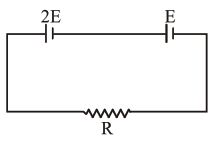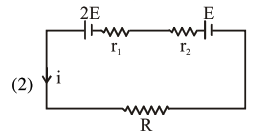# Two cells of emfQuestion:

Two cells of emf $2 \mathrm{E}$ and E with internal resistance $r_{1}$ and $r_{2}$ respectively are connected in series to an external resistor $R$ (see figure). The value of $R$, at which the potential difference across the terminals of the first cell becomes zero is1. $r_{1}+r_{2}$

2. $\frac{\mathrm{r}_{1}}{2}-\mathrm{r}_{2}$

3. $\frac{r_{1}}{2}+r_{2}$

4. $\mathrm{r}_{1}-\mathrm{r}_{2}$

Correct Option: , 2

Solution:$i=\frac{3 E}{R+r_{1}+r_{2}}$

$\mathrm{TPD}=2 \mathrm{E}-\mathrm{ir}_{1}=0$

$2 \mathrm{E}=\mathrm{ir}_{1}$

$2 \mathrm{E}=\frac{3 \mathrm{E} \times \mathrm{r}_{1}}{\mathrm{R}+\mathrm{r}_{1}+\mathrm{r}_{2}}$

$2 R+2 r_{1}+2 r_{2}=3 r_{1}$

$\mathrm{R}=\frac{\mathrm{r}_{1}}{2}-\mathrm{r}_{2}$